# Properties of Geometric Shapes & Angles Chapter Exam

Exam Instructions:

Choose your answers to the questions and click 'Next' to see the next set of questions. You can skip questions if you would like and come back to them later with the yellow "Go To First Skipped Question" button. When you have completed the practice exam, a green submit button will appear. Click it to see your results. Good luck!

Answered 0 of 30

### Page 1

#### Question 3 3. The isosceles triangle pictured below shows one angle measuring 40 degrees. What is the measure of angle x?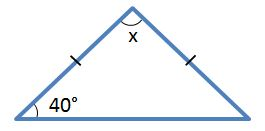#### Question 4 4. What type of triangle is pictured below?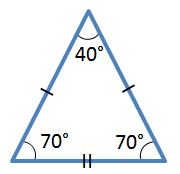#### Question 5 5. What is the perimeter of the pictured square?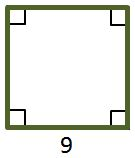### Page 2

#### Question 6 6. The pictured shape is a: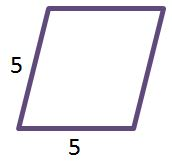#### Question 8 8. Study the given diagram. Find the value of x, the distance between T and E.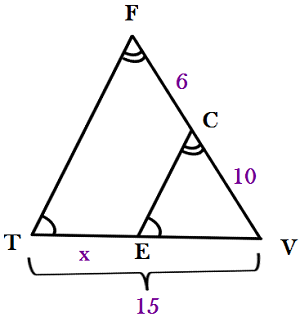#### Question 9 9. Consider the figure below, where Angle 4 = 12m - 4 Angle 5 = 10m + 20 Calculate the measure of Angle 5.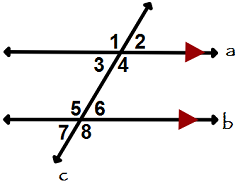#### Question 10 10. What is the relationship between Angle 2 and Angle 7?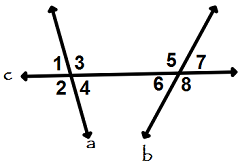### Page 3

#### Question 11 11. Determine if triangle VEZ is similar to triangle MAG.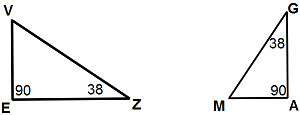#### Question 12 12. Is triangle ABC similar to triangle DEF?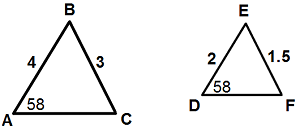#### Question 15 15. What is the line, as shown in the picture, called?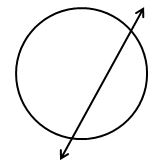### Page 4

#### Question 18 18. What is the area of the pictured rectangle?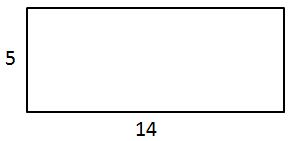#### Question 19 19. In the figure below, Angle 7 = 15x and Angle 10 = 9x + 30. Determine the measure of Angle 10.#### Question 20 20. In triangle GBN, Angle G is seven more than three times a number, Angle B is five more than four times the number, and Angle N is twelve less than five times the number. Solve for the measure of Angle G.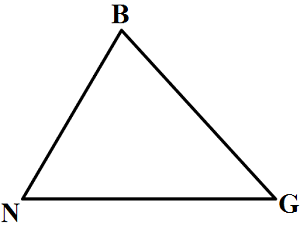### Page 5

#### Question 23 23. Which line is the transversal?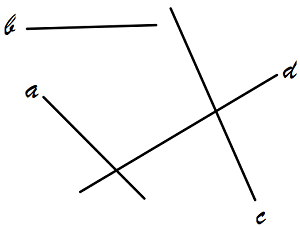### Page 6

#### Question 26 26. In the right triangle pictured below, what is the value of angle x?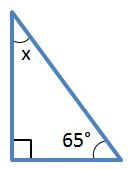#### Question 27 27. The pictured shape is a: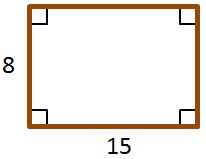#### Question 29 29. What is the relationship between Angle 1 and Angle 7?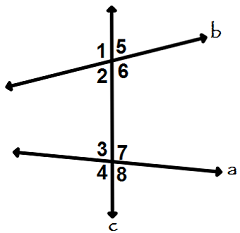#### Question 30 30. Mary claims that the triangles below are similar by AA. Do you agree or disagree with Mary?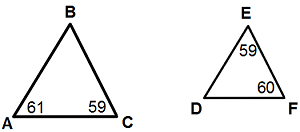#### Properties of Geometric Shapes & Angles Chapter Exam Instructions

Choose your answers to the questions and click 'Next' to see the next set of questions. You can skip questions if you would like and come back to them later with the yellow "Go To First Skipped Question" button. When you have completed the practice exam, a green submit button will appear. Click it to see your results. Good luck!

Support